1 篇文章 1 订阅

对于链接1里面是我最早看到的资料，前辈1在MATLAB中文论坛中发布自己搭建的工程文件及其所遇到的问题，后面楼主说是解决了问题，但是没有上传（这个可以谅解），另外里面有许多热心网友的回答是非常有用的（这些回答对我自己后面解决相关问题，成功实现Simulink仿真是非常关键的，在这里非常感谢论坛中的各位前辈）。

对于链接2这个确实是可以用的，只不过对MATLAB版本有限制。开始我下载之后用我的MATLAB2016b打开工程，点击run后就报错，The block 'BPPID/Subsystem/Unit Delay1' does not permit continuous sample time (0 or [0,0]) for the parameter 'SampleTime'.里面分了两大派，一部分人说亲测有效，大赞跟帖；一部分人也像我一样运行报错，此时我疑惑了。于是猜测可能是MATLAB版本问题，于是在另一台电脑上的MATLAB2014b版本运行，果然得到楼主那一条漂亮的曲线（虽然我的工作就是点击一下run，但是还是很开心）。但鉴于我的电脑安装的是MATLAB2016b版本，不可能让我卸载重装2014版吧。于是琢磨怎么将其移植到MATLAB2016b版本上。

对于链接3非常感谢这个楼主的S函数代码，里面注释很详细，我也是在这个楼主的基础上修改的（非常感谢）。接下来我将把我调试的整个流程做如下介绍：

1、编写S函数（感谢上面三位楼主，如有侵权，通知我撤销）

function [sys,x0,str,ts]=my_exppidf(t,x,u,flag)
switch flag,
case 0,
[sys,x0,str,ts]=mdlInitializeSizes;
case 2,
case 3,
sys=mdlOutputs(t,x,u);
case {1,4,9},
sys=[];
otherwise
error(['unhandled flag=',num2str(flag)]);%异常处理
end
function[sys,x0,str,ts]=mdlInitializeSizes
sizes=simsizes;%用于设置模块参数的结构体用simsizes来生成
sizes.NumContStates=0;%模块连续状态变量的个数
sizes.NumDiscStates=3;%模块离散状态变量的个数
sizes.NumOutputs=4;%模块输出变量的个数
sizes.NumInputs=7;%模块输入变量的个数
sizes.DirFeedthrough=1;%模块是否存在直接贯通，1表示存在直接贯通，若为0，则mdlOutputs函数里不能有u
sizes.NumSampleTimes=1;%模块的采样时间个数,至少是一个
sys=simsizes(sizes);%设置完后赋给sys输出
x0=zeros(3,1);%系统状态变量设置
str=[];
ts=[0 0];%采样周期设为0表示是连续系统，
%     ts=[0.001 0];%采样周期设为0表示是连续系统，
T=0.001;
x=[u(5);x(2)+u(5)*T;(u(5)-u(4))/T];%3个状态量（偏差、偏差和以及偏差变化量），u(5)是偏差，u(4)是上一次的偏差，x(2)则是之前的偏差和
sys=[x(1);x(2);x(3)];
function sys=mdlOutputs(t,x,u)
xite=0.2;
alfa=0.05;
IN=3;H=5;OUT=3;
wi=rand(5,3);%产生一个5*3的随机数矩阵，随机数在（0，1）区间
wi_1=wi;wi_2=wi;wi_3=wi;
wo=rand(3,5);
wo_1=wo;wo_2=wo;wo_3=wo;
Oh=zeros(5,1);%产生一个1*5的零矩阵(行矩阵)
I=Oh;
xi=[u(1),u(3),u(5)];%神经网络训练的3个输入，期望值、误差以及实际值
epid=[x(1);x(2);x(3)];%3个状态变量（偏差、偏差和、偏差变化量）（3*1矩阵，列向量）
I=xi*wi';%隐层的输入
for j=1:1:5
Oh(j)=(exp(I(j))-exp(-I(j)))/(exp(I(j))+exp(-I(j)));%隐层的输出值（1*5矩阵）行矩阵
end
K1=wo*Oh;%输出层的输入（3*1矩阵）
for i=1:1:3
K(i)=exp(K1(i))/(exp(K1(i))+exp(-K1(i)));%得到输出层的输出（KP、KI、KD）（1*3矩阵，行向量）
end
u_k=K*epid;%计算得到控制律u，1个值
%%以下是权值调整
%隐含层至输出层的权值调整
dyu=sign((u(3)-u(2))/(u(7)-u(6)+0.0001));
for j=1:1:3
dK(j)=2/(exp(K1(j))+exp(-K1(j)))^2; %输出层的输出的一阶导
end
for i=1:1:3
delta3(i)=u(5)*dyu*epid(i)*dK(i);  %输出层的delta
end
for j=1:1:3
for i=1:1:5
d_wo=xite*delta3(j)*Oh(i)+alfa*(wo_1-wo_2);
end
end
wo=wo_1+d_wo;
%以下是输入层至隐含层的权值调整
for i=1:1:5
dO(i)=4/(exp(I(i))+exp(-I(i)))^2;%(1*5矩阵)
end
segma=delta3*wo;%（1*5矩阵，行向量）
delta2 = dO.*segma;
d_wi = delta2'*xi+alfa*(wi_1-wi_2);
wi=wi_1+d_wi;
wo_3=wo_2;
wo_2=wo_1;
wo_1=wo;%储存输出层本次调整后的权值
wi_3=wi_2;
wi_2=wi_1;
wi_1=wi;%储存隐层本次调整后的权值
Kp=K(1);Ki=K(2);Kd=K(3);
sys=[u_k,Kp,Ki,Kd];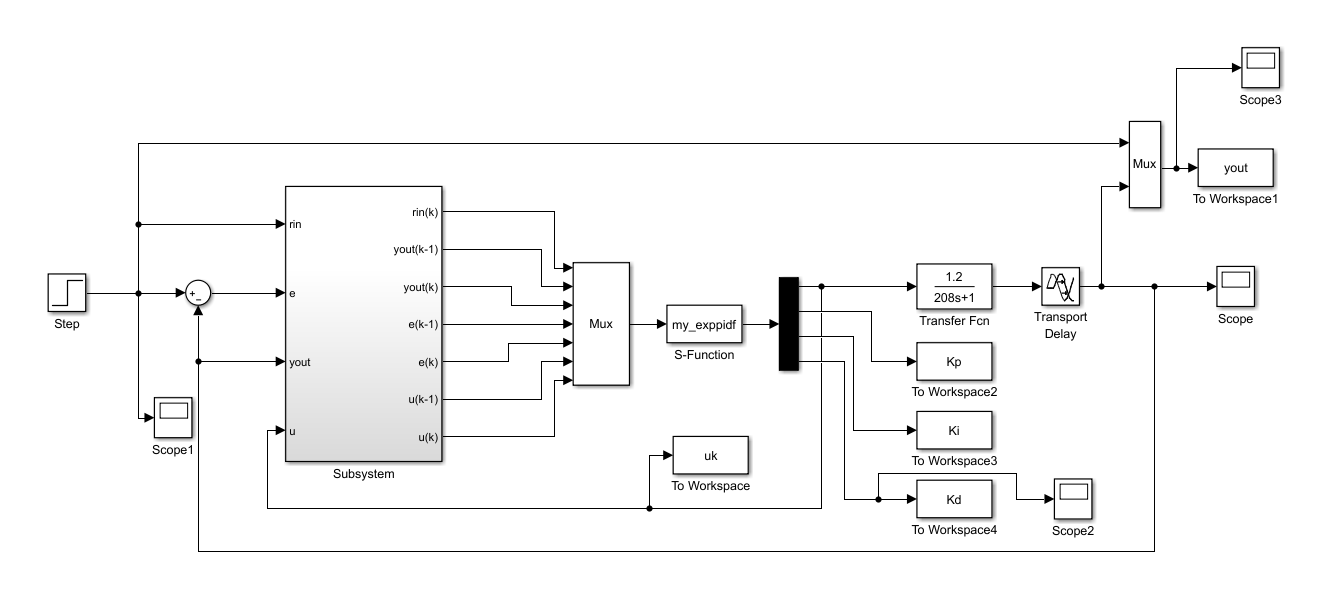其中Subsystem为：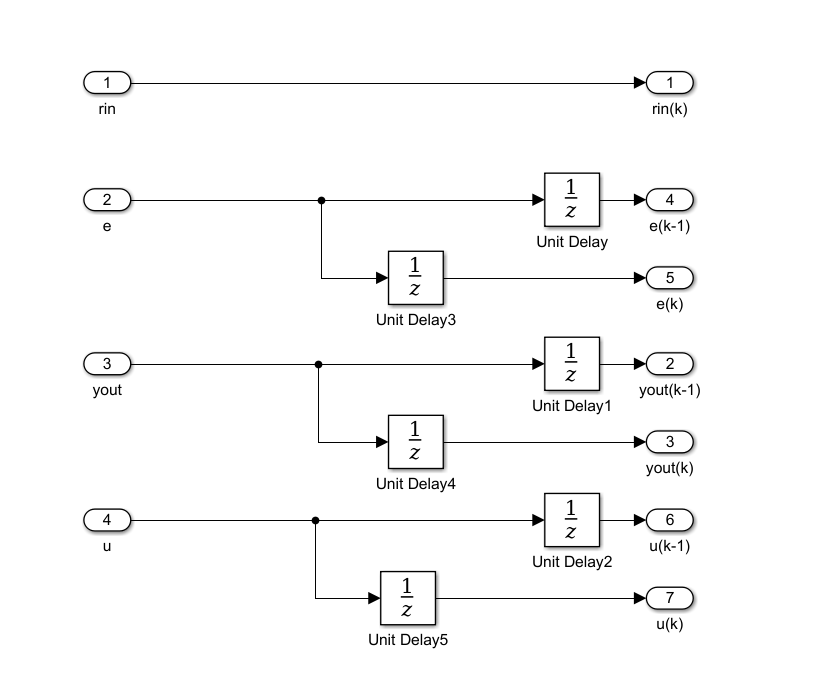3、相关参数配置

3.1 系统参数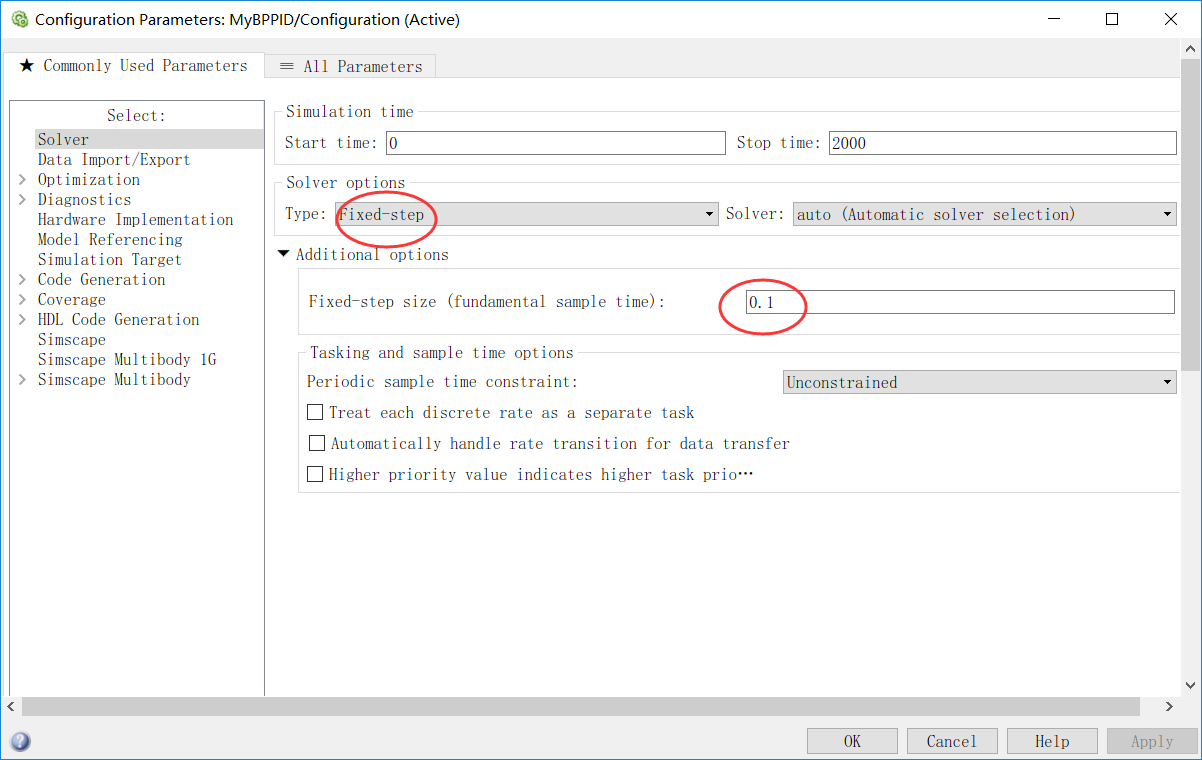3.2  Transport Delay 参数设置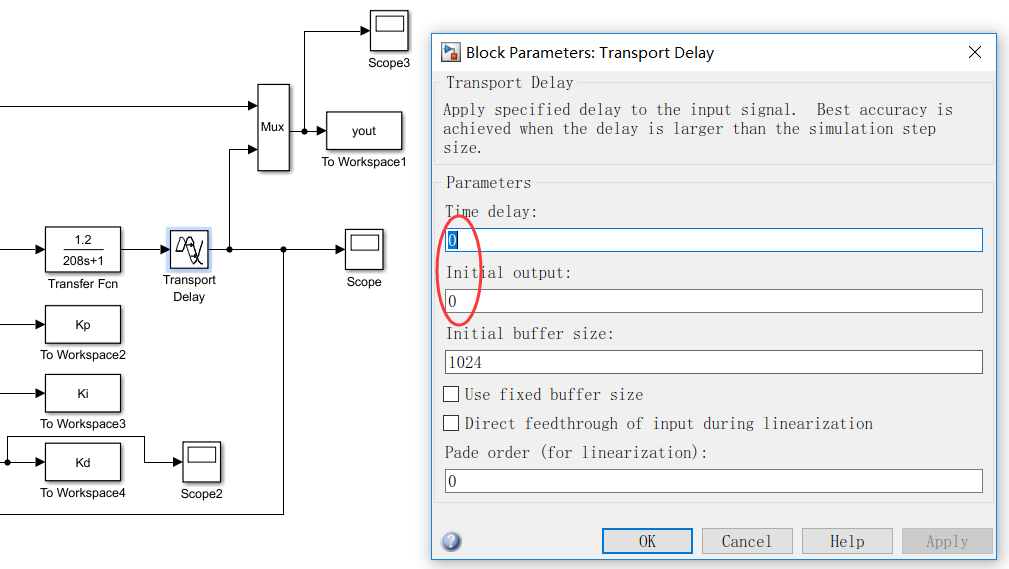3.3  Unit Delay 参数设置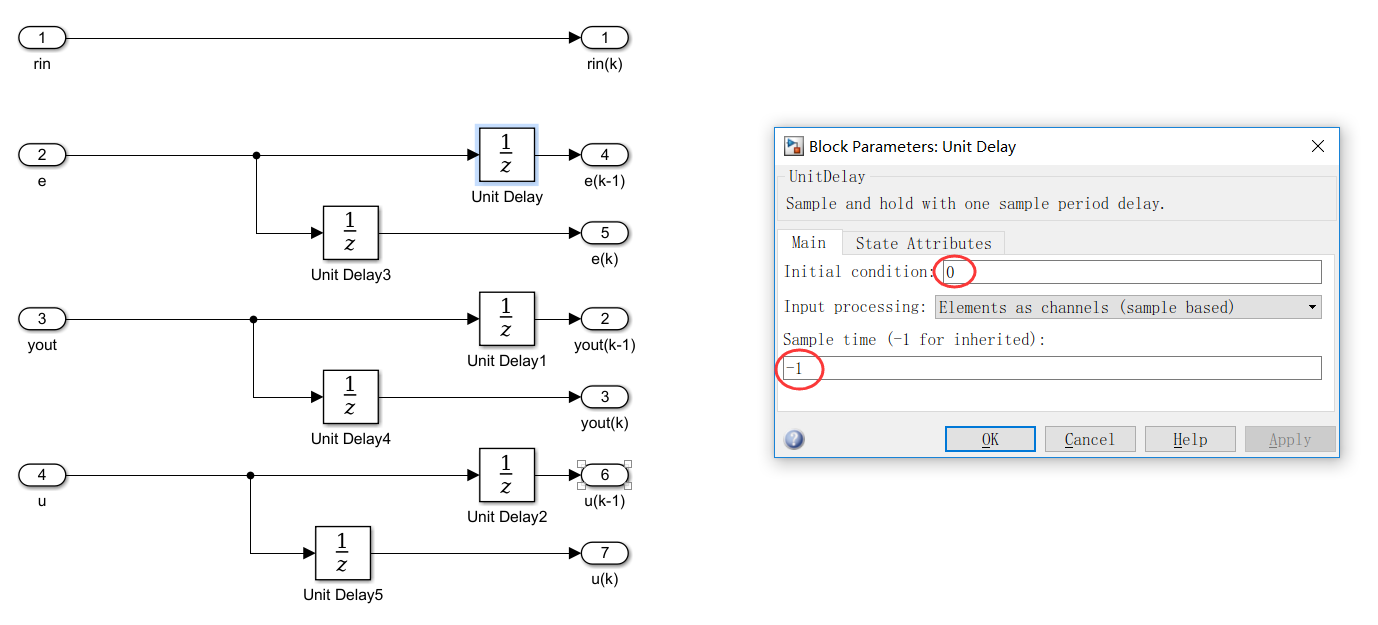tips：其中 系统参数fundamental sample time设置 与 Transport Delay 参数 Time delay 有联系，如果设置不好则会出现输出一直为0的情况。

4、实验结果

4.1 当fundamental sample time 设置为0.02时，实验结果如下：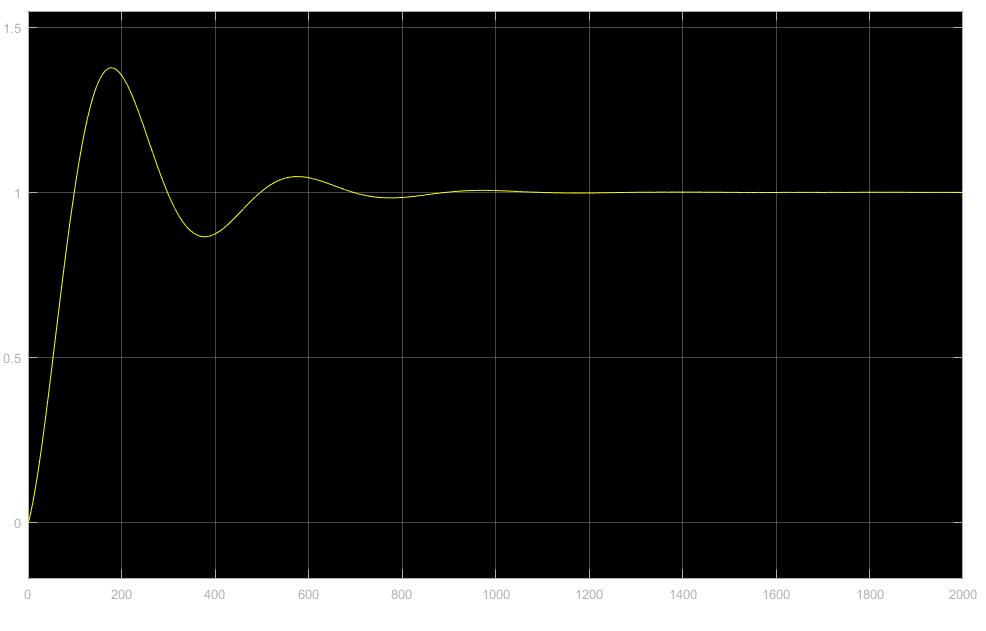4.2 当fundamental sample time 设置为0.1时，实验结果如下：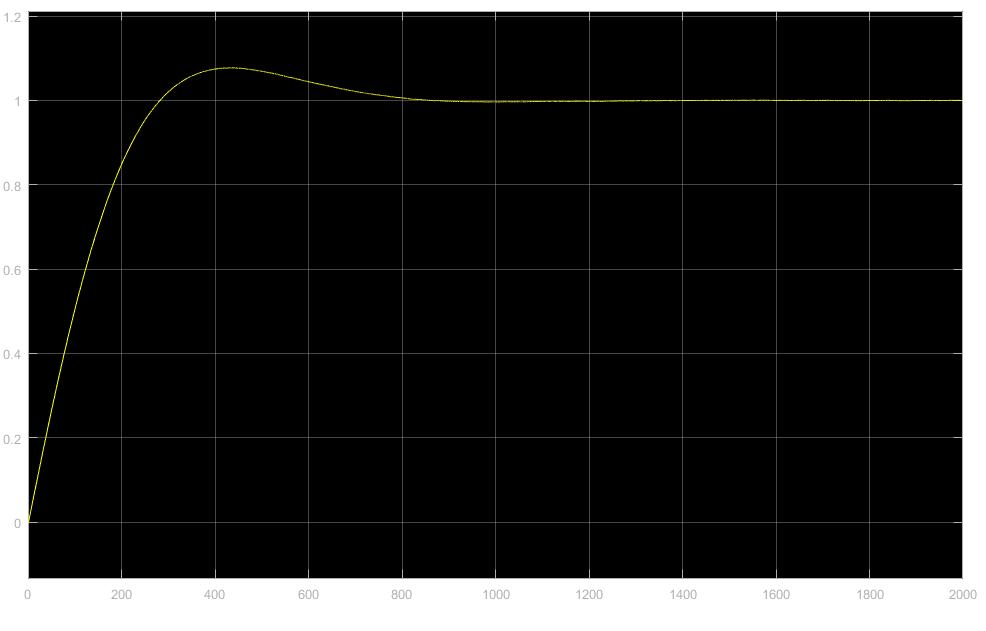01-06
11-2112万+
11-303324
11-273325
05-304万+
01-17528
01-31606
04-04
09-10
10-07点击重新获取扫码支付余额充值